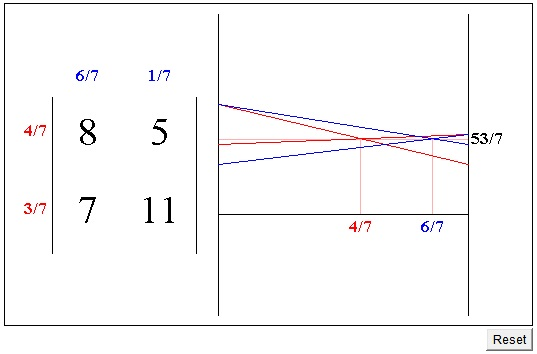# The Minimax Theorem

The applet below illustrates von Neumann's Minimax Theorem for two-person zero-sum games, where each of the players has a selection of two strategies. Such a game either has a saddle point or there is a stable combination of mixed strategies.

### If you are reading this, your browser is not set to run Java applets. Try IE11 or Safari and declare the site https://www.cut-the-knot.org as trusted in the Java setup.What if applet does not run?

(In the applet, the four matrix entries can be modified by clicking or by dragging next to the vertical center line of each of the numbers. The two vertical black lines on the right represent lines x = 0 and x = 1. The horizontal black line is the y-axis.)

Arrows, when appear, point from a larger to a smaller entry in a row, and from a smaller to a larger one in a column. Thus an entry pointed to in both its row and column is necessarily a saddle point. The proof of the existence of mixed maximin and minimax strategies in a 2×2 game is a direct consequence of Pappus' theorem. Playing with arrows reminded me of a trivial fact that has been used in one of the simplest problems I am aware of that may be proved with a minimum of practice and virtually no reference to a formal system: an arrow joining two points may point only in one of two directions. This fact has the following application: if a player has a dominant strategy, then the game has a saddle point. Indeed, if there is a dominant strategy for one of the players, then there are two parallel arrows pointing in the same direction. The arrow joining their endpoints points either to one or the other. The one that it points to is pointed to by two arrows and is therefore a saddle point.

Conversely, in the presence of a saddle point, one of the players is bound to have a dominant strategy, although a possibility of having equal matrix entries complicates somewhat the discussion and also the definitions.

To be continued ...

### References

1. A. Beck et al, Excursions into Mathematics, A K Peters, 2000
2. J. L. Casti, Five Golden Rules, John Wiley & Sons, 1996
3. For All Practical Purposes by COMAP, 6th edition, W. H. Freeman & Company, 2002
4. I. N. Herstein, I. Kaplansky, Matters Mathematical, Chelsea Publ, 1978
5. A. Rappoport, Two-Person Game Theory, Dover, 1966
6. P. D. Straffin, Game Theory and Strategy, MAA, 1993
7. A. Taylor, Mathematics and Politics, Springer, 1995